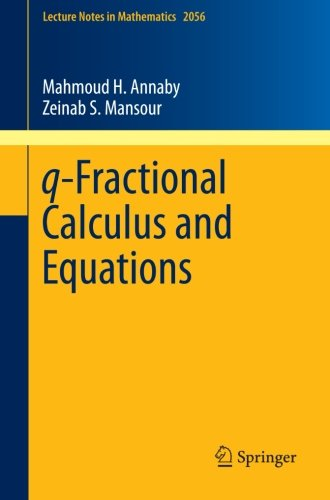# q-Fractional Calculus and Equations

• Length: 337 pages
• Edition: 1
• Publisher:
• Publication Date: 2012-08-26
• ISBN-10: 364230897X
• ISBN-13: 9783642308970
Description

This nine-chapter monograph introduces a rigorous investigation of q-difference operators in standard and fractional settings. It starts with elementary calculus of q-differences and integration of Jackson’s type before turning to q-difference equations. The existence and uniqueness theorems are derived using successive approximations, leading to systems of equations with retarded arguments. Regular  q-Sturm–Liouville theory is also introduced; Green’s function is constructed and the eigenfunction expansion theorem is given. The monograph also discusses some integral equations of Volterra and Abel type, as introductory material for the study of fractional q-calculi. Hence fractional q-calculi of the types Riemann–Liouville; Grünwald–Letnikov;  Caputo;  Erdélyi–Kober and Weyl are defined analytically. Fractional q-Leibniz rules with applications  in q-series are  also obtained with rigorous proofs of the formal  results of  Al-Salam-Verma, which remained unproved for decades. In working towards the investigation of q-fractional difference equations; families of q-Mittag-Leffler functions are defined and their properties are investigated, especially the q-Mellin–Barnes integral  and Hankel contour integral representation of  the q-Mittag-Leffler functions under consideration,  the distribution, asymptotic and reality of their zeros, establishing q-counterparts of Wiman’s results. Fractional q-difference equations are studied; existence and uniqueness theorems are given and classes of Cauchy-type problems are completely solved in terms of families of q-Mittag-Leffler functions. Among many q-analogs of classical results and concepts, q-Laplace, q-Mellin and q2-Fourier transforms are studied and their applications are investigated.